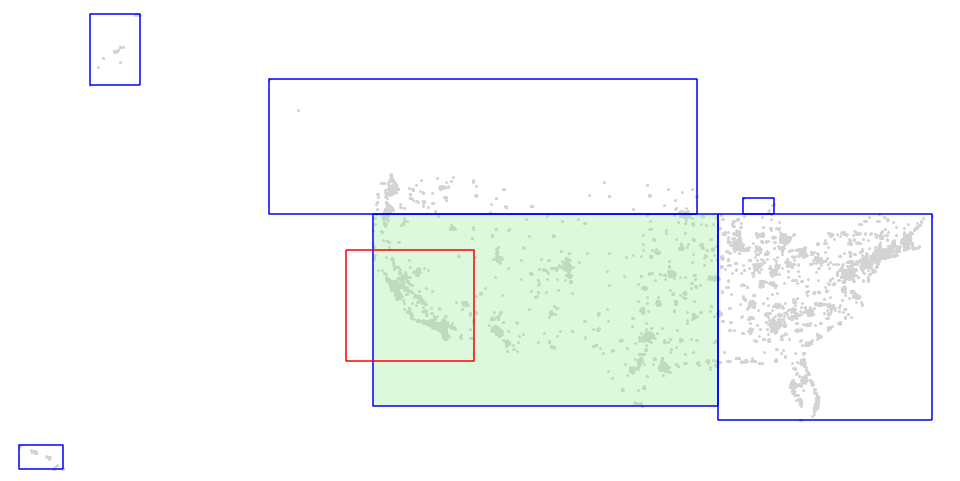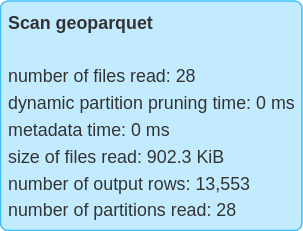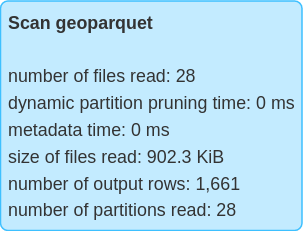# Query optimization

Sedona Spatial operators fully supports Apache SparkSQL query optimizer. It has the following query optimization features:

• Automatically optimizes range join query and distance join query.
• Automatically performs predicate pushdown.

Tip

Sedona join performance is heavily affected by the number of partitions. If the join performance is not ideal, please increase the number of partitions by doing `df.repartition(XXX)` right after you create the original DataFrame.

## Range join¶

Introduction: Find geometries from A and geometries from B such that each geometry pair satisfies a certain predicate. Most predicates supported by SedonaSQL can trigger a range join.

Spark SQL Example:

``````SELECT *
FROM polygondf, pointdf
WHERE ST_Contains(polygondf.polygonshape,pointdf.pointshape)
``````
``````SELECT *
FROM polygondf, pointdf
WHERE ST_Intersects(polygondf.polygonshape,pointdf.pointshape)
``````
``````SELECT *
FROM pointdf, polygondf
WHERE ST_Within(pointdf.pointshape, polygondf.polygonshape)
``````

Spark SQL Physical plan:

``````== Physical Plan ==
RangeJoin polygonshape#20: geometry, pointshape#43: geometry, false
:- Project [st_polygonfromenvelope(cast(_c0#0 as decimal(24,20)), cast(_c1#1 as decimal(24,20)), cast(_c2#2 as decimal(24,20)), cast(_c3#3 as decimal(24,20)), mypolygonid) AS polygonshape#20]
:  +- *FileScan csv
+- Project [st_point(cast(_c0#31 as decimal(24,20)), cast(_c1#32 as decimal(24,20)), myPointId) AS pointshape#43]
+- *FileScan csv
``````

Note

All join queries in SedonaSQL are inner joins

## Distance join¶

Introduction: Find geometries from A and geometries from B such that the distance of each geometry pair is less or equal than a certain distance. It supports the planar Euclidean distance calculator `ST_Distance` and the meter-based geodesic distance calculators `ST_DistanceSpheroid` and `ST_DistanceSphere`.

Spark SQL Example for planar Euclidean distance:

Only consider fully within a certain distance

``````SELECT *
FROM pointdf1, pointdf2
WHERE ST_Distance(pointdf1.pointshape1,pointdf2.pointshape2) < 2
``````

Consider intersects within a certain distance

``````SELECT *
FROM pointdf1, pointdf2
WHERE ST_Distance(pointdf1.pointshape1,pointdf2.pointshape2) <= 2
``````

Spark SQL Physical plan:

``````== Physical Plan ==
DistanceJoin pointshape1#12: geometry, pointshape2#33: geometry, 2.0, true
:- Project [st_point(cast(_c0#0 as decimal(24,20)), cast(_c1#1 as decimal(24,20)), myPointId) AS pointshape1#12]
:  +- *FileScan csv
+- Project [st_point(cast(_c0#21 as decimal(24,20)), cast(_c1#22 as decimal(24,20)), myPointId) AS pointshape2#33]
+- *FileScan csv
``````

Warning

If you use `ST_Distance` as the predicate, Sedona doesn't control the distance's unit (degree or meter). It is same with the geometry. If your coordinates are in the longitude and latitude system, the unit of `distance` should be degree instead of meter or mile. To change the geometry's unit, please either transform the coordinate reference system to a meter-based system. See ST_Transform. If you don't want to transform your data, please consider using `ST_DistanceSpheroid` or `ST_DistanceSphere`.

Spark SQL Example for meter-based geodesic distance `ST_DistanceSpheroid` (works for `ST_DistanceSphere` too):

Less than a certain distance==

``````SELECT *
FROM pointdf1, pointdf2
WHERE ST_DistanceSpheroid(pointdf1.pointshape1,pointdf2.pointshape2) < 2
``````

Less than or equal to a certain distance==

``````SELECT *
FROM pointdf1, pointdf2
WHERE ST_DistanceSpheroid(pointdf1.pointshape1,pointdf2.pointshape2) <= 2
``````

Warning

If you use `ST_DistanceSpheroid` or `ST_DistanceSphere` as the predicate, the unit of the distance is meter. Currently, distance join with geodesic distance calculators work best for point data. For non-point data, it only considers their centroids. The distance join algorithm internally uses an approximate distance buffer which might lead to inaccurate results if your data is close to the poles or antimeridian.

Introduction: Perform a range join or distance join but broadcast one of the sides of the join. This maintains the partitioning of the non-broadcast side and doesn't require a shuffle.

Sedona will create a spatial index on the broadcasted table.

Sedona uses broadcast join only if the correct side has a broadcast hint. The supported join type - broadcast side combinations are:

• Inner - either side, preferring to broadcast left if both sides have the hint
• Left semi - broadcast right
• Left anti - broadcast right
• Left outer - broadcast right
• Right outer - broadcast left
``````pointDf.alias("pointDf").join(broadcast(polygonDf).alias("polygonDf"), expr("ST_Contains(polygonDf.polygonshape, pointDf.pointshape)"))
``````

Spark SQL Physical plan:

``````== Physical Plan ==
BroadcastIndexJoin pointshape#52: geometry, BuildRight, BuildRight, false ST_Contains(polygonshape#30, pointshape#52)
:- Project [st_point(cast(_c0#48 as decimal(24,20)), cast(_c1#49 as decimal(24,20))) AS pointshape#52]
:  +- FileScan csv
+- SpatialIndex polygonshape#30: geometry, QUADTREE, [id=#62]
+- Project [st_polygonfromenvelope(cast(_c0#22 as decimal(24,20)), cast(_c1#23 as decimal(24,20)), cast(_c2#24 as decimal(24,20)), cast(_c3#25 as decimal(24,20))) AS polygonshape#30]
+- FileScan csv
``````

This also works for distance joins with `ST_Distance`, `ST_DistanceSpheroid` or `ST_DistanceSphere`:

``````pointDf1.alias("pointDf1").join(broadcast(pointDf2).alias("pointDf2"), expr("ST_Distance(pointDf1.pointshape, pointDf2.pointshape) <= 2"))
``````

Spark SQL Physical plan:

``````== Physical Plan ==
BroadcastIndexJoin pointshape#52: geometry, BuildRight, BuildLeft, true, 2.0 ST_Distance(pointshape#52, pointshape#415) <= 2.0
:- Project [st_point(cast(_c0#48 as decimal(24,20)), cast(_c1#49 as decimal(24,20))) AS pointshape#52]
:  +- FileScan csv
+- SpatialIndex pointshape#415: geometry, QUADTREE, [id=#1068]
+- Project [st_point(cast(_c0#48 as decimal(24,20)), cast(_c1#49 as decimal(24,20))) AS pointshape#415]
+- FileScan csv
``````

Note: If the distance is an expression, it is only evaluated on the first argument to ST_Distance (`pointDf1` above).

When one table involved a spatial join query is smaller than a threadhold, Sedona will automatically choose broadcast index join instead of Sedona optimized join. The current threshold is controlled by sedona.join.autoBroadcastJoinThreshold and set to the same as `spark.sql.autoBroadcastJoinThreshold`.

## Google S2 based approximate equi-join¶

If the performance of Sedona optimized join is not ideal, which is possibly caused by complicated and overlapping geometries, you can resort to Sedona built-in Google S2-based approximate equi-join. This equi-join leverages Spark's internal equi-join algorithm and might be performant given that you can opt to skip the refinement step by sacrificing query accuracy.

### 1. Generate S2 ids for both tables¶

Use ST_S2CellIds to generate cell IDs. Each geometry may produce one or more IDs.

``````SELECT id, geom, name, explode(ST_S2CellIDs(geom, 15)) as cellId
FROM lefts
``````
``````SELECT id, geom, name, explode(ST_S2CellIDs(geom, 15)) as cellId
FROM rights
``````

### 2. Perform equi-join¶

Join the two tables by their S2 cellId

``````SELECT lcs.id as lcs_id, lcs.geom as lcs_geom, lcs.name as lcs_name, rcs.id as rcs_id, rcs.geom as rcs_geom, rcs.name as rcs_name
FROM lcs JOIN rcs ON lcs.cellId = rcs.cellId
``````

### 3. Optional: Refine the result¶

Due to the nature of S2 Cellid, the equi-join results might have a few false-positives depending on the S2 level you choose. A smaller level indicates bigger cells, less exploded rows, but more false positives.

To ensure the correctness, you can use one of the Spatial Predicates to filter out them. Use this query instead of the query in Step 2.

``````SELECT lcs.id as lcs_id, lcs.geom as lcs_geom, lcs.name as lcs_name, rcs.id as rcs_id, rcs.geom as rcs_geom, rcs.name as rcs_name
FROM lcs, rcs
WHERE lcs.cellId = rcs.cellId AND ST_Contains(lcs.geom, rcs.geom)
``````

As you see, compared to the query in Step 2, we added one more filter, which is `ST_Contains`, to remove false positives. You can also use `ST_Intersects` and so on.

Tip

You can skip this step if you don't need 100% accuracy and want faster query speed.

### 4. Optional: De-duplcate¶

Due to the explode function used when we generate S2 Cell Ids, the resulting DataFrame may have several duplicate matches. You can remove them by performing a GroupBy query.

``````SELECT lcs_id, rcs_id , first(lcs_geom), first(lcs_name), first(rcs_geom), first(rcs_name)
FROM joinresult
GROUP BY (lcs_id, rcs_id)
``````

The `first` function is to take the first value from a number of duplicate values.

If you don't have a unique id for each geometry, you can also group by geometry itself. See below:

``````SELECT lcs_geom, rcs_geom, first(lcs_name), first(rcs_name)
FROM joinresult
GROUP BY (lcs_geom, rcs_geom)
``````

Note

If you are doing point-in-polygon join, this is not a problem and you can safely discard this issue. This issue only happens when you do polygon-polygon, polygon-linestring, linestring-linestring join.

### S2 for distance join¶

This also works for distance join. You first need to use `ST_Buffer(geometry, distance)` to wrap one of your original geometry column. If your original geometry column contains points, this `ST_Buffer` will make them become circles with a radius of `distance`.

Since the coordinates are in the longitude and latitude system, so the unit of `distance` should be degree instead of meter or mile. You can get an approximation by performing `METER_DISTANCE/111000.0`, then filter out false-positives. Note that this might lead to inaccurate results if your data is close to the poles or antimeridian.

In a nutshell, run this query first on the left table before Step 1. Please replace `METER_DISTANCE` with a meter distance. In Step 1, generate S2 IDs based on the `buffered_geom` column. Then run Step 2, 3, 4 on the original `geom` column.

``````SELECT id, geom , ST_Buffer(geom, METER_DISTANCE/111000.0) as buffered_geom, name
FROM lefts
``````

## Regular spatial predicate pushdown¶

Introduction: Given a join query and a predicate in the same WHERE clause, first executes the Predicate as a filter, then executes the join query.

Spark SQL Example:

``````SELECT *
FROM polygondf, pointdf
WHERE ST_Contains(polygondf.polygonshape,pointdf.pointshape)
AND ST_Contains(ST_PolygonFromEnvelope(1.0,101.0,501.0,601.0), polygondf.polygonshape)
``````

Spark SQL Physical plan:

``````== Physical Plan ==
RangeJoin polygonshape#20: geometry, pointshape#43: geometry, false
:- Project [st_polygonfromenvelope(cast(_c0#0 as decimal(24,20)), cast(_c1#1 as decimal(24,20)), cast(_c2#2 as decimal(24,20)), cast(_c3#3 as decimal(24,20)), mypolygonid) AS polygonshape#20]
:  +- Filter  **org.apache.spark.sql.sedona_sql.expressions.ST_Contains\$**
:     +- *FileScan csv
+- Project [st_point(cast(_c0#31 as decimal(24,20)), cast(_c1#32 as decimal(24,20)), myPointId) AS pointshape#43]
+- *FileScan csv
``````

## Push spatial predicates to GeoParquet¶

Sedona supports spatial predicate push-down for GeoParquet files. When spatial filters were applied to dataframes backed by GeoParquet files, Sedona will use the `bbox` properties in the metadata to determine if all data in the file will be discarded by the spatial predicate. This optimization could reduce the number of files scanned when the queried GeoParquet dataset was partitioned by spatial proximity.

To maximize the performance of Sedona GeoParquet filter pushdown, we suggest that you sort the data by their geohash values (see ST_GeoHash) and then save as a GeoParquet file. An example is as follows:

``````SELECT col1, col2, geom, ST_GeoHash(geom, 5) as geohash
FROM spatialDf
ORDER BY geohash
``````

The following figure is the visualization of a GeoParquet dataset. `bbox`es of all GeoParquet files were plotted as blue rectangles and the query window was plotted as a red rectangle. Sedona will only scan 1 of the 6 files to answer queries such as `SELECT * FROM geoparquet_dataset WHERE ST_Intersects(geom, <query window>)`, thus only part of the data covered by the light green rectangle needs to be scanned.We can compare the metrics of querying the GeoParquet dataset with or without the spatial predicate and observe that querying with spatial predicate results in fewer number of rows scanned.

Without spatial predicate With spatial predicateLast update: May 31, 2023 08:01:37